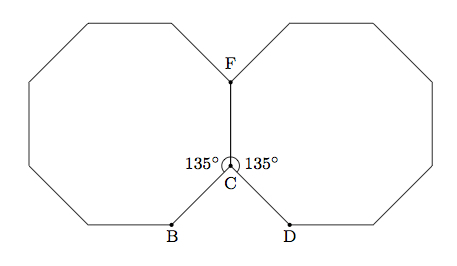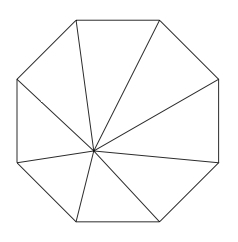# Tile Patterns I: octagons and squares

Alignments to Content Standards: 8.G.A.5

One interesting tile pattern (see for example this Tile Pattern) has four octagons surrounding what appears to be a square as in the picture below:The pattern can then be extended up, down, to the left, and to the right.

1. Suppose each octagon is a regular octagon, that is an octagon where all $8$ sides are congruent and all $8$ angles are congruent. Find the measure of the $8$ interior angles.
2. Show that the space between the four octagons, shaded in the picture, is in fact a square.

## IM Commentary

Tiles and tiling patterns are good sources for developing geometric intuition. This task aims at explaining why four regular octagons can be placed around a central square, applying student knowledge of triangles and sums of angles in both triangles and more general polygons. There are two steps to the problem, corresponding to the two vital aspects of a square. First, the $4$ sides must be congruent: this comes from the fact that the regular octagons enclosing the square are congruent. Then the $4$ angles must be right angles and this takes more care to show.

Perhaps the most common shape of tiles used for covering two dimensional surfaces is squares. Squares fit nicely together in a grid which can be extended as far as desired:Regular hexagons are also relatively common are considered in the task ''Tiling patterns II: Hexagons.'' Students may be encouraged to develop their own patterns of polygons which can be used to tile the plane.

While aspects of this task might be used for assessment, the task is ideally suited for instruction purposes as the mathematical content is directly related to, but somewhat exceeds, the content of standard 8.G.5 on sums of angles in triangles. This task could profitably used to foster growth with respect to the standards for mathematical practice: Like its companion ''Tile patterns II: hexagons'', it encourages students to engage in MP2 (reason abstractly and quantitatively) and MP3 (construct viable arguments).

## Solutions

Solution: Measuring Turning Angles

1. Suppose you are travelling counterclockwise along the octagon. At each corner, you make a turn. After $8$ of these turns you are heading in the same direction in which you started and have made one full revolution of $360^\circ$. Since the angles in the regular octagon are all congruent, each of these turns must be $\frac{360^\circ}{8} = 45^\circ$. Since the sum of the interior angle and $45^\circ$ is $180^\circ$, this means that the interior angle must measure $135^\circ$:In the picture, the interior angle is angle $ABC$ while the $45^\circ$ ''turn'' is angle $PBC$.

2. The picture below includes a second octagon adjacent to the first:We know that $$m(\angle BCD) + m(\angle BCF) + m(\angle DCF) = 360$$ because these three angles make a full circle. Since $m(\angle BCF) = m(\angle DCF) = 135$ from part (a) we conclude that $m(\angle BCD) = 90$. The same argument, repeated for the other pairs of adjacent octagons in the configuration, show that all four angles in the green quadrilateral are right angles. All four sides are congruent as well because they are sides of congruent regular octagons. This show that the green quadrilateral bounded by the four octagons is a square.

Solution: 2 Triangulating the octagon (alternate solution to part a)

We can divide the octagon into triangles by adding a vertex on the interior as pictured below:The vertex could be at the center but does not need to be for the argument. The picture shows that the octagon is divided into $8$ triangles. The sum of the angles in each of the triangles is $180^\circ$. So adding up all of the angles of the eight triangles gives $8 \times 180^\circ$. All of these angles give the $8$ interior angles of the octagon together with the circle around the interior vertex. This circle has $360$ degrees and so we find $$\text{Sum of Interior Angles of Octagon measure } 8 \times 180^\circ - 360^\circ.$$ The eight interior angles of the octagon are congruent since it is a regular octagon and so we finally get $$\text{Interior Angle of Octagon measures} \frac{8 \times 180^\circ - 360^\circ}{8} = 135^\circ.$$